• 导数公式微分公式积分公式的比较,将同类函数的导数、微分和积分公式放在一起，一目了然，加强理解记忆。
• Matlab 的 Matlab微分和导数MATLAB提供用于计算符号导数的diff命令。 以最简单的形式，将要微分的功能传递给diff命令作为参数。例如，计算函数的导数的方程式 -例子创建脚本文件并在其中键入以下代码 -syms tf = 3*t...

Matlab 的 Matlab微分和导数
MATLAB提供用于计算符号导数的diff命令。 以最简单的形式，将要微分的功能传递给diff命令作为参数。
例如，计算函数的导数的方程式 -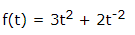例子
创建脚本文件并在其中键入以下代码 -
syms t
f = 3*t^2 + 2*t^(-2);
diff(f)
执行上面示例代码，得到以下结果 -
Trial>> syms t
f = 3*t^2 + 2*t^(-2);
diff(f)
ans =
6*t - 4/t^3
以下是使用Octave 计算的写法 -
symbols
t = sym("t");
f = 3*t^2 + 2*t^(-2);
differentiate(f,t)
执行上面示例代码，得到以下结果 -
ans =
6*t - 4/t^3
基本微分规则的验证
下面简要说明微分规则的各种方程或规则，并验证这些规则。 为此，我们将写一个第一阶导数f'(x)和二阶导数f“(x)。
以下是微分的规则 -
规则 - 1
对于任何函数f和g，任何实数a和b是函数的导数：
h(x) = af(x) + bg(x)相对于x，由h’(x) = af’(x) + bg’(x)给出。
规则 - 2
sum和subtraction规则表述为：如果f和g是两个函数，则f'和g'分别是它们的导数，如下 -
(f + g)' = f' + g'
(f - g)' = f' - g'
规则 - 3
product规则表述为：如果f和g是两个函数，则f'和g'分别是它们的导数，如下 -
(f.g)' = f'.g + g'.f
规则 - 4
quotient规则表明，如果f和g是两个函数，则f'和g'分别是它们的导数，那么 -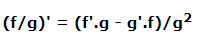规则 - 5
多项式或基本次幂规则表述为：如果y = f(x)= x^n，则 -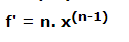这个规则的直接结果是任何常数的导数为零，即如果y = k，那么为任何常数 -
f' = 0
规则 - 5
chain规则表述为 - 相对于x的函数h(x)= f(g(x))的函数的导数是 -
h'(x)= f'(g(x)).g'(x)
例子
创建脚本文件并在其中键入以下代码 -
syms x
syms t
f = (x + 2)*(x^2 + 3)
der1 = diff(f)
f = (t^2 + 3)*(sqrt(t) + t^3)
der2 = diff(f)
f = (x^2 - 2*x + 1)*(3*x^3 - 5*x^2 + 2)
der3 = diff(f)
f = (2*x^2 + 3*x)/(x^3 + 1)
der4 = diff(f)
f = (x^2 + 1)^17
der5 = diff(f)
f = (t^3 + 3* t^2 + 5*t -9)^(-6)
der6 = diff(f)
执行上面示例代码，得到 以下结果 -
f =
(x^2 + 3)*(x + 2)
der1 =
2*x*(x + 2) + x^2 + 3
f =
(t^(1/2) + t^3)*(t^2 + 3)
der2 =
(t^2 + 3)*(3*t^2 + 1/(2*t^(1/2))) + 2*t*(t^(1/2) + t^3)
f =
(x^2 - 2*x + 1)*(3*x^3 - 5*x^2 + 2)
der3 =
(2*x - 2)*(3*x^3 - 5*x^2 + 2) - (- 9*x^2 + 10*x)*(x^2 - 2*x + 1)
f =
(2*x^2 + 3*x)/(x^3 + 1)
der4 =
(4*x + 3)/(x^3 + 1) - (3*x^2*(2*x^2 + 3*x))/(x^3 + 1)^2
f =
(x^2 + 1)^17
der5 =
34*x*(x^2 + 1)^16
f =
1/(t^3 + 3*t^2 + 5*t - 9)^6
der6 =
-(6*(3*t^2 + 6*t + 5))/(t^3 + 3*t^2 + 5*t - 9)^7
以下是对上面示例的Octave写法 -
symbols
x=sym("x");
t=sym("t");
f = (x + 2)*(x^2 + 3)
der1 = differentiate(f,x)
f = (t^2 + 3)*(t^(1/2) + t^3)
der2 = differentiate(f,t)
f = (x^2 - 2*x + 1)*(3*x^3 - 5*x^2 + 2)
der3 = differentiate(f,x)
f = (2*x^2 + 3*x)/(x^3 + 1)
der4 = differentiate(f,x)
f = (x^2 + 1)^17
der5 = differentiate(f,x)
f = (t^3 + 3* t^2 + 5*t -9)^(-6)
der6 = differentiate(f,t)
指数，对数和三角函数的导数
下表提供了常用指数，对数和三角函数的导数，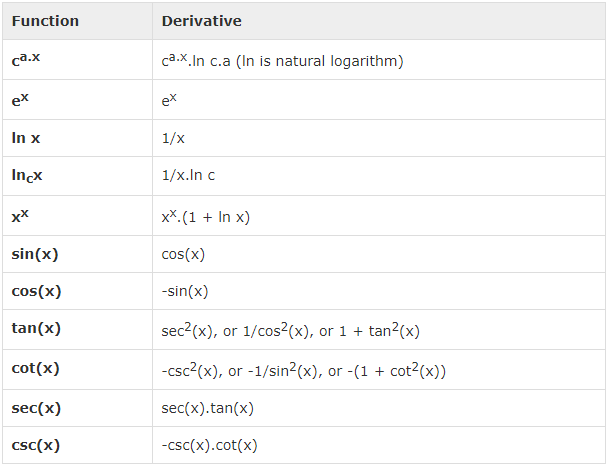例子
创建脚本文件并在其中键入以下代码 -
syms x
y = exp(x)
diff(y)
y = x^9
diff(y)
y = sin(x)
diff(y)
y = tan(x)
diff(y)
y = cos(x)
diff(y)
y = log(x)
diff(y)
y = log10(x)
diff(y)
y = sin(x)^2
diff(y)
y = cos(3*x^2 + 2*x + 1)
diff(y)
y = exp(x)/sin(x)
diff(y)
执行上面示例代码，得到以下结果 -
y =
exp(x)
ans =
exp(x)
y =
x^9
ans =
9*x^8
y =
sin(x)
ans =
cos(x)
y =
tan(x)
ans =
tan(x)^2 + 1
y =
cos(x)
ans =
-sin(x)
y =
log(x)
ans =
1/x
y =
log(x)/log(10)
ans =
1/(x*log(10))
y =
sin(x)^2
ans =
2*cos(x)*sin(x)
y =
cos(3*x^2 + 2*x + 1)
ans =
-sin(3*x^2 + 2*x + 1)*(6*x + 2)
y =
exp(x)/sin(x)
ans =
exp(x)/sin(x) - (exp(x)*cos(x))/sin(x)^2
以下代码是上面代码的Octave写法 -
symbols
x = sym("x");
y = Exp(x)
differentiate(y,x)
y = x^9
differentiate(y,x)
y = Sin(x)
differentiate(y,x)
y = Tan(x)
differentiate(y,x)
y = Cos(x)
differentiate(y,x)
y = Log(x)
differentiate(y,x)
% symbolic packages does not have this support
%y = Log10(x)
%differentiate(y,x)
y = Sin(x)^2
differentiate(y,x)
y = Cos(3*x^2 + 2*x + 1)
differentiate(y,x)
y = Exp(x)/Sin(x)
differentiate(y,x)
计算高阶导数
要计算函数f的较高导数，可使用diff(f，n)。
计算函数的二阶导数公式为 -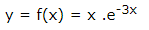f = x*exp(-3*x);
diff(f, 2)
MATLAB执行上面代码将返回以下结果 -
ans =
9*x*exp(-3*x) - 6*exp(-3*x)
以下是使用Octave重写上面示例，代码如下 -
symbols
x = sym("x");
f = x*Exp(-3*x);
differentiate(f, x, 2)
例子
在这个例子中，要解决一个问题。由给定函数y = f(x)= 3sin(x)+ 7cos(5x)，来找出方程f“+ f = -5cos(2x)是否成立。
创建脚本文件并在其中键入以下代码 -
syms x
y = 3*sin(x)+7*cos(5*x); % defining the function
lhs = diff(y,2)+y; %evaluting the lhs of the equation
rhs = -5*cos(2*x); %rhs of the equation
if(isequal(lhs,rhs))
disp('Yes, the equation holds true');
else
disp('No, the equation does not hold true');
end
disp('Value of LHS is: '), disp(lhs);
运行文件时，会显示以下结果 -
No, the equation does not hold true
Value of LHS is:
-168*cos(5*x)
以上是上面示例的Octave写法 -
symbols
x = sym("x");
y = 3*Sin(x)+7*Cos(5*x); % defining the function
lhs = differentiate(y, x, 2) + y; %evaluting the lhs of the equation
rhs = -5*Cos(2*x); %rhs of the equation
if(lhs == rhs)
disp('Yes, the equation holds true');
else
disp('No, the equation does not hold true');
end
disp('Value of LHS is: '), disp(lhs);
查找曲线的最大和最小值
如果正在搜索图形的局部最大值和最小值，基本上是在特定地点的函数图上或符号变量的特定值范围内查找最高点或最低点。
对于函数y = f(x)，图形具有零斜率的图上的点称为固定点。 换句话说，固定点是f'(x)= 0。
要找到微分的函数的固定点，需要将导数设置为零并求解方程。
示例
要找到函数f(x)= 2x3 + 3x2 - 12x + 17的固定点
可参考以下步骤 -
首先输入函数并绘制图，代码如下 -
syms x
y = 2*x^3 + 3*x^2 - 12*x + 17; % defining the function
ezplot(y)
执行上面示例代码，得到以下结果 -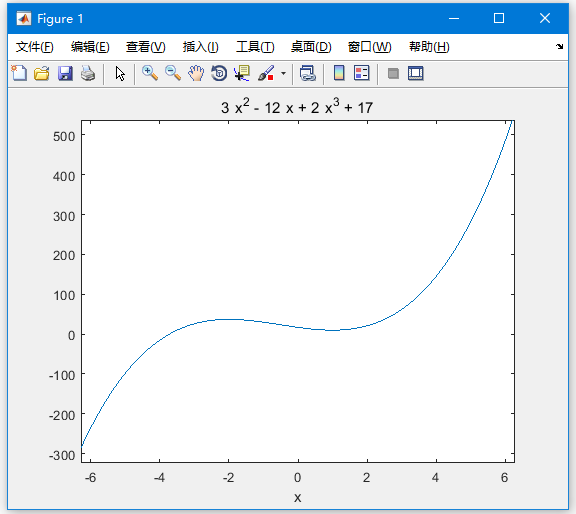以上是上面示例的Octave写法 -
symbols
x = sym('x');
y = inline("2*x^3 + 3*x^2 - 12*x + 17");
ezplot(y)
print -deps graph.eps
我们的目标是在图上找到一些局部最大值和最小值，假设要找到图中间隔在[-2,2]的局部最大值和最小值。参考以下示例代码 -
syms x
y = 2*x^3 + 3*x^2 - 12*x + 17; % defining the function
ezplot(y, [-2, 2])
执行上面示例代码，得到以下结果 -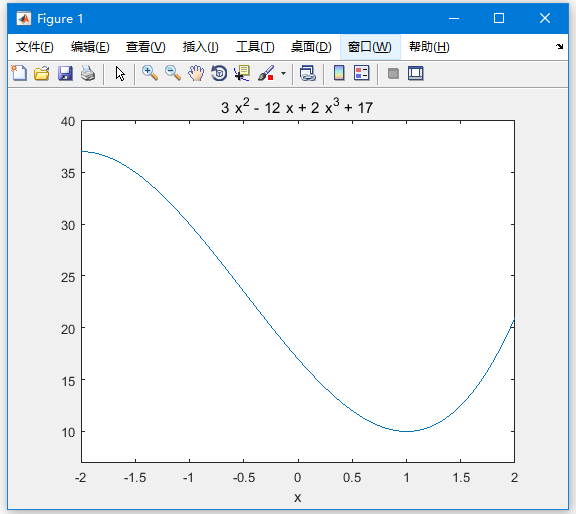以下是上面示例的Octave写法 -
symbols
x = sym('x');
y = inline("2*x^3 + 3*x^2 - 12*x + 17");
ezplot(y, [-2, 2])
print -deps graph.eps
接下来，需要计算导数。
g = diff(y)
MATLAB执行代码并返回以下结果 -
g =
6*x^2 + 6*x - 12
以下是上面示例的Octave写法 -
symbols
x = sym("x");
y = 2*x^3 + 3*x^2 - 12*x + 17;
g = differentiate(y,x)
接下来求解导数函数g，得到它变为零的值。
s = solve(g)
MATLAB执行代码并返回以下结果 -
s =
1
-2
以下是上面示例的Octave写法 -
symbols
x = sym("x");
y = 2*x^3 + 3*x^2 - 12*x + 17;
g = differentiate(y,x)
roots([6, 6, -12])
这与我们设想情节一致。 因此，要评估临界点x = 1，-2处的函数f。可以使用subs命令替换符号函数中的值。
subs(y, 1), subs(y, -2)
MATLAB执行代码并返回以下结果 -
ans =
10
ans =
37
以下是上面示例的Octave写法 -
symbols
x = sym("x");
y = 2*x^3 + 3*x^2 - 12*x + 17;
g = differentiate(y,x)
roots([6, 6, -12])
subs(y, x, 1), subs(y, x, -2)
因此，在间隔[-2,2]中函数f(x)= 2x^3 + 3x^2 - 12x + 17的最小值和最大值分别为10和37。
求解微分方程
MATLAB提供了用于求解微分方程的dsolve命令。
找到单个方程的解的最基本的dsolve命令形式是 -
dsolve('eqn')
其中eqn是用于输入方程式的文本串。
它返回一个符号解，其中包含一组任意常量，MATLAB标记C1，C2等等。
还可以为问题指定初始和边界条件，以逗号分隔的列表遵循以下公式：
dsolve('eqn','cond1', 'cond2',…)
为了使用dsolve命令，导数用D表示。例如，像f'(t)= -2 * f + cost(t)这样的等式输入为 -
'Df = -2*f + cos(t)'
较高阶导数由D导数的顺序表示。
例如，方程f"(x) + 2f'(x) = 5sin3x应输入为 -
'D2y + 2Dy = 5*sin(3*x)'
下面来看一个一阶微分方程的简单例子：y'= 5y。
s = dsolve('Dy = 5*y')
MATLAB执行代码并返回以下结果 -
s =
C2*exp(5*t)
再来一个二阶微分方程的例子：y“-y = 0，y(0)= -1，y'(0)= 2。
dsolve('D2y - y = 0','y(0) = -1','Dy(0) = 2')
MATLAB执行代码并返回以下结果 -
ans =
exp(t)/2 - (3*exp(-t))/2

展开全文• 导数公式微分公式积分公式大全 感谢观看 期待关注 知乎：叄贰壹 简书：带只拖鞋去流浪 关注我，带你一起写bug warning ：未经授权，不得转载 有问题的小伙伴请在下方留言，喜欢就点个赞吧 ...
高等数学导数公式、微分公式和积分公式大全感谢观看 期待关注知乎：叄贰壹
简书：带只拖鞋去流浪
关注我，带你一起写bug
warning ：未经授权，不得转载
有问题的小伙伴请在下方留言，喜欢就点个赞吧
展开全文高等数学 机器学习
• ## MATLAB微分和导数

千次阅读 2019-01-24 10:14:40
以最简单的形式，将要微分的功能传递给diff命令作为参数。例如，计算函数的导数的方程式 - 例子创建脚本文件并在其中键入以下代码 -syms t f = 3t^2 + 2t^(-2); diff(f) MATLAB执行上面示例代码，得到以下结果 - ...
MATLAB提供用于计算符号导数的diff命令。 以最简单的形式，将要微分的功能传递给diff命令作为参数。例如，计算函数的导数的方程式 - 例子创建脚本文件并在其中键入以下代码 -syms t f = 3t^2 + 2t^(-2); diff(f) MATLAB执行上面示例代码，得到以下结果 - Trial>> syms t f = 3t^2 + 2t^(-2); diff(f)
ans =
6*t - 4/t^3 Shell以下是使用Octave 计算的写法 -pkg load symbolic symbols
t = sym(“t”); f = 3t^2 + 2t^(-2); differentiate(f,t) MATLAB执行上面示例代码，得到以下结果 - ans =
6*t - 4/t^3 Shell基本微分规则的验证下面简要说明微分规则的各种方程或规则，并验证这些规则。 为此，我们将写一个第一阶导数f’(x)和二阶导数f“(x)。以下是微分的规则 -规则 - 1对于任何函数f和g，任何实数a和b是函数的导数：h(x) = af(x) + bg(x)相对于x，由h’(x) = af’(x) + bg’(x)给出。规则 - 2sum和subtraction规则表述为：如果f和g是两个函数，则f’和g’分别是它们的导数，如下 - (f + g)’ = f’ + g’
(f - g)’ = f’ - g’ 规则 - 3product规则表述为：如果f和g是两个函数，则f’和g’分别是它们的导数，如下 - (f.g)’ = f’.g + g’.f 规则 - 4quotient规则表明，如果f和g是两个函数，则f’和g’分别是它们的导数，那么 -规则 - 5多项式或基本次幂规则表述为：如果y = f(x)= x^n，则 - 这个规则的直接结果是任何常数的导数为零，即如果y = k，那么为任何常数 - f’ = 0 规则 - 5chain规则表述为 - 相对于x的函数h(x)= f(g(x))的函数的导数是 - h’(x)= f’(g(x)).g’(x) MATLAB例子 创建脚本文件并在其中键入以下代码 -syms x syms t f = (x + 2)(x^2 + 3) der1 = diff(f) f = (t^2 + 3)(sqrt(t) + t^3) der2 = diff(f) f = (x^2 - 2x + 1)(3x^3 - 5x^2 + 2) der3 = diff(f) f = (2x^2 + 3x)/(x^3 + 1) der4 = diff(f) f = (x^2 + 1)^17 der5 = diff(f) f = (t^3 + 3* t^2 + 5t -9)^(-6) der6 = diff(f) MATLAB执行上面示例代码，得到 以下结果 - f = (x^2 + 3)(x + 2)
der1 = 2x(x + 2) + x^2 + 3
f = (t^(1/2) + t3)*(t2 + 3)
der2 = (t^2 + 3)(3t^2 + 1/(2t^(1/2))) + 2t*(t^(1/2) + t^3)
f = (x^2 - 2x + 1)(3x^3 - 5x^2 + 2)
der3 = (2x - 2)(3x^3 - 5x^2 + 2) - (- 9x^2 + 10x)(x^2 - 2x + 1)
f = (2x^2 + 3x)/(x^3 + 1)
der4 = (4x + 3)/(x^3 + 1) - (3x2*(2*x2 + 3*x))/(x^3 + 1)^2
f = (x^2 + 1)^17
der5 = 34x(x^2 + 1)^16
f = 1/(t^3 + 3t^2 + 5t - 9)^6
der6 = -(6*(3t^2 + 6t + 5))/(t^3 + 3t^2 + 5t - 9)^7 Shell以下是对上面示例的Octave写法 -pkg load symbolic symbols x=sym(“x”); t=sym(“t”); f = (x + 2)(x^2 + 3) der1 = differentiate(f,x) f = (t^2 + 3)(t^(1/2) + t^3) der2 = differentiate(f,t) f = (x^2 - 2x + 1)(3x^3 - 5x^2 + 2) der3 = differentiate(f,x) f = (2x^2 + 3x)/(x^3 + 1) der4 = differentiate(f,x) f = (x^2 + 1)^17 der5 = differentiate(f,x) f = (t^3 + 3* t^2 + 5t -9)^(-6) der6 = differentiate(f,t) MATLAB指数，对数和三角函数的导数下表提供了常用指数，对数和三角函数的导数，例子 创建脚本文件并在其中键入以下代码 -syms x y = exp(x) diff(y) y = x^9 diff(y) y = sin(x) diff(y) y = tan(x) diff(y) y = cos(x) diff(y) y = log(x) diff(y) y = log10(x) diff(y) y = sin(x)^2 diff(y) y = cos(3x^2 + 2*x + 1) diff(y) y = exp(x)/sin(x) diff(y) MATLAB执行上面示例代码，得到以下结果 - y = exp(x) ans = exp(x)
y = x^9 ans = 9*x^8
y = sin(x) ans = cos(x)
y = tan(x) ans = tan(x)^2 + 1
y = cos(x) ans = -sin(x)
y = log(x) ans = 1/x
y = log(x)/log(10) ans = 1/(x*log(10))
y = sin(x)^2 ans = 2*cos(x)*sin(x)
y =
cos(3x^2 + 2x + 1) ans = -sin(3x^2 + 2x + 1)(6x + 2)
y = exp(x)/sin(x) ans = exp(x)/sin(x) - (exp(x)*cos(x))/sin(x)^2 Shell以下代码是上面代码的Octave写法 - pkg load symbolic symbols
x = sym(“x”); y = Exp(x) differentiate(y,x)
y = x^9 differentiate(y,x)
y = Sin(x) differentiate(y,x)
y = Tan(x) differentiate(y,x)
y = Cos(x) differentiate(y,x)
y = Log(x) differentiate(y,x)
% symbolic packages does not have this support %y = Log10(x) %differentiate(y,x)
y = Sin(x)^2 differentiate(y,x)
y = Cos(3x^2 + 2x + 1) differentiate(y,x)
y = Exp(x)/Sin(x) differentiate(y,x) Shell计算高阶导数要计算函数f的较高导数，可使用diff(f，n)。 计算函数的二阶导数公式为 - f = xexp(-3x); diff(f, 2) MATLAB MATLAB执行上面代码将返回以下结果 - ans = 9xexp(-3x) - 6exp(-3*x) Shell 以下是使用Octave重写上面示例，代码如下 - pkg load symbolic symbols
x = sym(“x”); f = xExp(-3x);
differentiate(f, x, 2) MATLAB 例子 在这个例子中，要解决一个问题。由给定函数y = f(x)= 3sin(x)+ 7cos(5x)，来找出方程f“+ f = -5cos(2x)是否成立。 创建脚本文件并在其中键入以下代码 - syms x y = 3sin(x)+7cos(5x); % defining the function lhs = diff(y,2)+y; %evaluting the lhs of the equation rhs = -5cos(2x); %rhs of the equation if(isequal(lhs,rhs)) disp(‘Yes, the equation holds true’); else disp(‘No, the equation does not hold true’); end disp('Value of LHS is: '), disp(lhs); MATLAB 运行文件时，会显示以下结果 - No, the equation does not hold true Value of LHS is: -168cos(5*x) Shell 以上是上面示例的Octave写法 - pkg load symbolic symbols
x = sym(“x”); y = 3Sin(x)+7Cos(5x); % defining the function lhs = differentiate(y, x, 2) + y; %evaluting the lhs of the equation rhs = -5Cos(2*x); %rhs of the equation
if(lhs == rhs) disp(‘Yes, the equation holds true’); else disp(‘No, the equation does not hold true’); end disp('Value of LHS is: ‘), disp(lhs); MATLAB 查找曲线的最大和最小值如果正在搜索图形的局部最大值和最小值，基本上是在特定地点的函数图上或符号变量的特定值范围内查找最高点或最低点。 对于函数y = f(x)，图形具有零斜率的图上的点称为固定点。 换句话说，固定点是f’(x)= 0。 要找到微分的函数的固定点，需要将导数设置为零并求解方程。 示例 要找到函数f(x)= 2x3 + 3x2 - 12x + 17的固定点 可参考以下步骤 - 首先输入函数并绘制图，代码如下 - syms x y = 2x^3 + 3x^2 - 12*x + 17; % defining the function ezplot(y) MATLAB 执行上面示例代码，得到以下结果 - 以上是上面示例的Octave写法 - pkg load symbolic symbols
x = sym(‘x’); y = inline(“2x^3 + 3x^2 - 12*x + 17”);
ezplot(y) print -deps graph.eps MATLAB 我们的目标是在图上找到一些局部最大值和最小值，假设要找到图中间隔在[-2,2]的局部最大值和最小值。参考以下示例代码 - syms x y = 2x^3 + 3x^2 - 12*x + 17; % defining the function ezplot(y, [-2, 2]) MATLAB 执行上面示例代码，得到以下结果 - 以下是上面示例的Octave写法 - pkg load symbolic symbols
x = sym(‘x’); y = inline(“2x^3 + 3x^2 - 12*x + 17”);
ezplot(y, [-2, 2]) print -deps graph.eps MATLAB 接下来，需要计算导数。 g = diff(y) MATLAB MATLAB执行代码并返回以下结果 - g =
6x^2 + 6x - 12 Shell 以下是上面示例的Octave写法 - pkg load symbolic symbols
x = sym(“x”);
y = 2x^3 + 3x^2 - 12*x + 17; g = differentiate(y,x) MATLAB 接下来求解导数函数g，得到它变为零的值。 s = solve(g) MATLAB MATLAB执行代码并返回以下结果 - s = 1 -2 Shell 以下是上面示例的Octave写法 - pkg load symbolic symbols
x = sym(“x”);
y = 2x^3 + 3x^2 - 12*x + 17; g = differentiate(y,x) roots([6, 6, -12]) MATLAB 这与我们设想情节一致。 因此，要评估临界点x = 1，-2处的函数f。可以使用subs命令替换符号函数中的值。 subs(y, 1), subs(y, -2) MATLAB MATLAB执行代码并返回以下结果 - ans = 10 ans = 37 Shell 以下是上面示例的Octave写法 - pkg load symbolic symbols
x = sym(“x”);
y = 2x^3 + 3x^2 - 12*x + 17; g = differentiate(y,x)
roots([6, 6, -12])
subs(y, x, 1), subs(y, x, -2) MATLAB 因此，在间隔[-2,2]中函数f(x)= 2x^3 + 3x^2 - 12x + 17的最小值和最大值分别为10和37。 求解微分方程MATLAB提供了用于求解微分方程的dsolve命令。 找到单个方程的解的最基本的dsolve命令形式是 - dsolve(‘eqn’) MATLAB 其中eqn是用于输入方程式的文本串。 它返回一个符号解，其中包含一组任意常量，MATLAB标记C1，C2等等。 还可以为问题指定初始和边界条件，以逗号分隔的列表遵循以下公式： dsolve(‘eqn’,‘cond1’, ‘cond2’,…) 为了使用dsolve命令，导数用D表示。例如，像f’(t)= -2 * f + cost(t)这样的等式输入为 - ‘Df = -2f + cos(t)’ 较高阶导数由D导数的顺序表示。 例如，方程f"(x) + 2f’(x) = 5sin3x应输入为 - 'D2y + 2Dy = 5sin(3x)’ 下面来看一个一阶微分方程的简单例子：y’= 5y。 s = dsolve('Dy = 5y’) MATLAB执行代码并返回以下结果 - s = C2exp(5t) Shell 再来一个二阶微分方程的例子：y“-y = 0，y(0)= -1，y’(0)= 2。 dsolve(‘D2y - y = 0’,‘y(0) = -1’,‘Dy(0) = 2’) MATLAB MATLAB执行代码并返回以下结果 - ans = exp(t)/2 - (3*exp(-t))/2@TOC
欢迎使用Markdown编辑器
你好！ 这是你第一次使用 Markdown编辑器 所展示的欢迎页。如果你想学习如何使用Markdown编辑器, 可以仔细阅读这篇文章，了解一下Markdown的基本语法知识。
新的改变
我们对Markdown编辑器进行了一些功能拓展与语法支持，除了标准的Markdown编辑器功能，我们增加了如下几点新功能，帮助你用它写博客：
全新的界面设计 ，将会带来全新的写作体验；在创作中心设置你喜爱的代码高亮样式，Markdown 将代码片显示选择的高亮样式 进行展示；增加了 图片拖拽 功能，你可以将本地的图片直接拖拽到编辑区域直接展示；全新的 KaTeX数学公式 语法；增加了支持甘特图的mermaid语法1 功能；增加了 多屏幕编辑 Markdown文章功能；增加了 焦点写作模式、预览模式、简洁写作模式、左右区域同步滚轮设置 等功能，功能按钮位于编辑区域与预览区域中间；增加了 检查列表 功能。
功能快捷键
撤销：Ctrl/Command + Z 重做：Ctrl/Command + Y 加粗：Ctrl/Command + B 斜体：Ctrl/Command + I 标题：Ctrl/Command + Shift + H 无序列表：Ctrl/Command + Shift + U 有序列表：Ctrl/Command + Shift + O 检查列表：Ctrl/Command + Shift + C 插入代码：Ctrl/Command + Shift + K 插入链接：Ctrl/Command + Shift + L 插入图片：Ctrl/Command + Shift + G
合理的创建标题，有助于目录的生成
直接输入1次#，并按下space后，将生成1级标题。 输入2次#，并按下space后，将生成2级标题。 以此类推，我们支持6级标题。有助于使用TOC语法后生成一个完美的目录。
如何改变文本的样式
强调文本 强调文本
加粗文本 加粗文本
标记文本
删除文本

引用文本

H2O is是液体。
210 运算结果是 1024.
插入链接与图片
图片:带尺寸的图片:居中的图片:居中并且带尺寸的图片:当然，我们为了让用户更加便捷，我们增加了图片拖拽功能。
如何插入一段漂亮的代码片
去博客设置页面，选择一款你喜欢的代码片高亮样式，下面展示同样高亮的 代码片.
// An highlighted block
var foo = 'bar';

生成一个适合你的列表
项目
项目
项目
项目1项目2项目3
计划任务 完成任务
创建一个表格
一个简单的表格是这么创建的：
项目Value电脑$1600手机$12导管\$1
设定内容居中、居左、居右
使用:---------:居中 使用:----------居左 使用----------:居右
第一列第二列第三列第一列文本居中第二列文本居右第三列文本居左
SmartyPants
SmartyPants将ASCII标点字符转换为“智能”印刷标点HTML实体。例如：
TYPEASCIIHTMLSingle backticks'Isn't this fun?'‘Isn’t this fun?’Quotes"Isn't this fun?"“Isn’t this fun?”Dashes-- is en-dash, --- is em-dash– is en-dash, — is em-dash
创建一个自定义列表

Markdown

Text-to-
HTML conversion tool

Authors

John

Luke

如何创建一个注脚
一个具有注脚的文本。2
注释也是必不可少的
Markdown将文本转换为 HTML。
KaTeX数学公式
您可以使用渲染LaTeX数学表达式 KaTeX:
Gamma公式展示

Γ

(

n

)

=

(

n

−

1

)

!

∀

n

∈

N

是通过欧拉积分

Γ

(

z

)

=

∫

0

∞

t

z

−

1

e

−

t

d

t
&ThinSpace;

.

\Gamma(z) = \int_0^\infty t^{z-1}e^{-t}dt\,.

你可以找到更多关于的信息 LaTeX 数学表达式here.

新的甘特图功能，丰富你的文章

Mon 06

Mon 13

Mon 20

已完成

进行中

计划一

计划二

现有任务

Adding GANTT diagram functionality to mermaid

关于 甘特图 语法，参考 这儿,
UML 图表
可以使用UML图表进行渲染。 Mermaid. 例如下面产生的一个序列图：:

张三

李四

王五

你好！李四, 最近怎么样?

你最近怎么样，王五？

我很好，谢谢!

我很好，谢谢!

李四想了很长时间,

文字太长了

不适合放在一行.

打量着王五...

很好... 王五, 你怎么样?

张三

李四

王五

这将产生一个流程图。:

链接

长方形

圆

圆角长方形

菱形

关于 Mermaid 语法，参考 这儿,
FLowchart流程图
我们依旧会支持flowchart的流程图：

Created with Raphaël 2.2.0

开始

我的操作

确认？

结束

yes

no

关于 Flowchart流程图 语法，参考 这儿.
导出与导入
导出
如果你想尝试使用此编辑器, 你可以在此篇文章任意编辑。当你完成了一篇文章的写作, 在上方工具栏找到 文章导出 ，生成一个.md文件或者.html文件进行本地保存。
导入
如果你想加载一篇你写过的.md文件或者.html文件，在上方工具栏可以选择导入功能进行对应扩展名的文件导入， 继续你的创作。

mermaid语法说明 ↩︎ 注脚的解释 ↩︎

展开全文MATLAB
• 解法1：利用微分求解。 △f=f(x)△x+o(△x)=df+o(△x)。因为o(△x)趋于无穷小所以忽略不计。于是有△f≈df。 所以f(x+△x)≈f(x)+f(x)△x。本质上是函数局部线性化。 解法2：利用导数求解。 根据导数定义有f(x...
例题
计算cos29°的近似值。
解答过程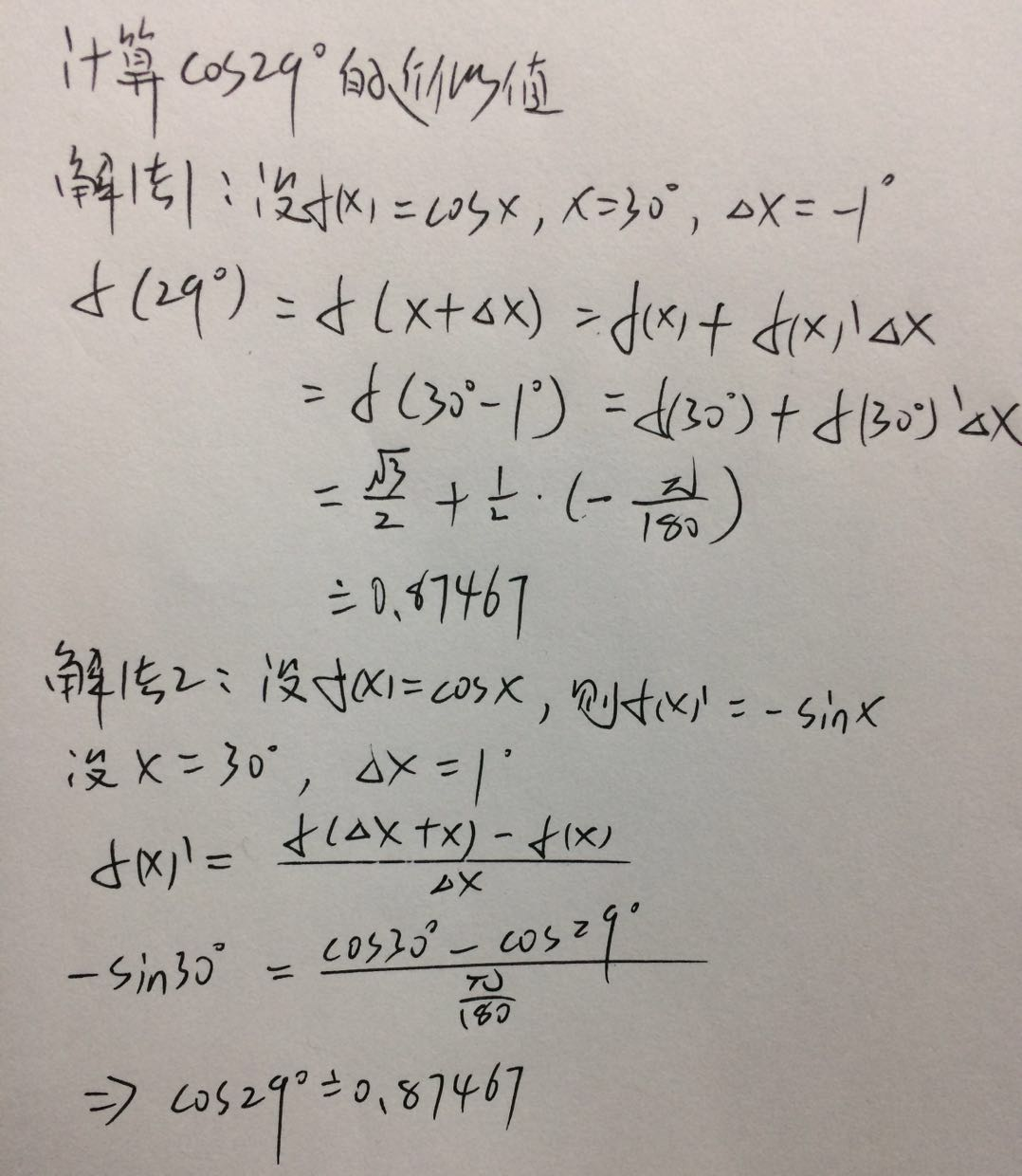分析
解法1：利用微分求解。
△f=f(x)△x+o(△x)=df+o(△x)。因为o(△x)趋于无穷小所以忽略不计。于是有△f≈df。
所以f(x+△x)≈f(x)+f(x)△x。本质上是函数局部线性化。

解法2：利用导数求解。
根据导数定义有f(x)=[f(x+△x)-f(x)]/△x或者f(x1)=[f(x1)-f(x2)]/(x1-x2)。

展开全文• 本文会详细的定义微分，并结合导数的概念，详细的说明二者的差别。...而微分的定义为当x发生微小变化时，在y方向上发生的微小变化，由导数公式我们来看 ，则可以有则有， 则最终有： （式一） 其...
• 这几天突然想到了优化理论中的梯度下降算法，看到了几个名词，愣了一下，虽然...数学中的全微分(方程)，全导数(公式)，偏微分(方程)，梯度，导数，方向导数，切线，斜率，射线，可导与连续，(多元)函数的积分与微分,...
• 1.求导法则(u±v)′=u′±v′(u\pm v)'=u'\pm v' (uv)′=u′v+v′u(uv)'=u'v+v'u (uv)′=u′v−v′uv2( \frac u v)'...常用公式(xa)′=axa−1(x^a)'=ax^{a-1} (ax)′=axlna(a^x)'=a^xlna (sinx)′=cosx(\sin x)'=\c机器学习
• 学习到机器学习线性回归逻辑回归时遇到了梯度下降算法，然后顺着扯出了一堆高数的相关概念理论：导数、偏导数、全微分、方向导数、梯度，重新回顾它们之间的一些关系，从网上教材中摘录相关知识点。 通过函数...高等数学 机器学习 梯度
• ## 微分和积分数学公式大全

万次阅读 多人点赞 2018-01-06 11:23:15
一、极限公式 （系数不为0的情况） 二、重要公式 三、下列常用等价无穷小关系（x->0） ...五、基本导数公式 ...七、基本初等函数的n阶导数公式高等数学
• 1. 基本性质 Tr(A)=Tr(AT)\text{Tr}(\mathrm A)=\text{Tr}(\mathrm A^T) Tr(ABC)=Tr(BCA)=Tr(CAB)\text{Tr}(\mathrm {ABC})=\text{Tr}(\mathrm {BCA})=\text{...因此如果 A\mathrm A C\mathrm C 互逆的话，三者相乘
• 导数微分常用公式(基础) 一。导数的定义； 1.导数的定义： 导数其实就是函数某点附近的 0000\frac{0}{0} 型极限 f′(x)=limh→0f(x+h)−f(x)hf′(x)=limh→0f(x+h)−f(x)hf'(x)=\lim_{h \to 0}\frac{f(x+h)-f...
• 一、极限公式 ...七、基本初等函数的n阶导数公式 八、微分公式与微分运算法则 九、微分运算法则 十、基本积分公式 十一、下列常用凑微分公式 十二、补充下面几个积分公式 十三...
• ## 微分和积分公式大全

万次阅读 多人点赞 2019-05-02 11:29:39
4 基本导数公式 5 高阶导数的运算法则 6 基本初等函数的n阶导数公式 7 微分公式与微分运算法则 8 微分运算法则 9 基本积分公式 10 下列常用凑微分公式 11 补充下面几个积分公式 12 分部积分法公式 13 第二...
• 3.微分导数关系 4.偏导 类比于一元函数，也想研究函数的变化率问题，在日常生活中，我们经常遇到这样的问题，一个值许多元素相关，我们习惯只改变一个变量值，其它变量值固定，看变化的情况。 这
• ## 导数与积分公式

千次阅读 2020-10-28 08:51:33
4 基本导数公式 5 高阶导数的运算法则 6 基本初等函数的n阶导数公式 7 微分公式与微分运算法则 8 微分运算法则 9 基本积分公式 10 下列常用凑微分公式 11 补充下面几个积分公式 12 分部积分...
• ## 导数与微分

千次阅读 2017-02-21 22:36:13
导数的定义：  当函数 y=f(x) 的自变量 x 在一点 x0 上产生一个增量 Δx 时，函数输出值的增量 Δy 与自变量... 导数公式定义为： 导数的意义：  物理意义： 表示运动物体瞬时速度 即增量 Δy 除以自变量 Δx ：
•数学
• 文章目录基本导数公式基本导数运算法则加法运算减法运算乘法运算除法运算带有常数C的导数微分的四则运算加减法计算带有常数的微分乘法计算除法计算 基本导数公式 原函数f(x)f(x)f(x) 导数f′(x)f'(x)f′(x) ...初等数学
• 目录简化版教程知乎马同学教程 简化版教程 该教程提出导数的方向概念，导数可以认为是向量。但该教程没有考虑到方向导数射线的性质。 （转）导数、偏导数、方向导数、梯度、...微分和导数微分导数 梯度 ...
• ## 高数积分导数公式

万次阅读 2018-06-05 23:49:13
积分公式汇总导数公式：导数&amp;微分微积分有两种定义： 1、古典微积分 这是一种直观、便于理解的定义。首先定义微分是微小变化量。比如函数y=f(x)中dx是x的微小变化量，那么dy就是dx对应的y的微小变化。导数...高数积分
• 自己整理的导数公式及积分公式记忆方法 常用的导数公式，俗称的16个 基本的积分公式，14个 两者对应的记忆：（以导数公式作为参照，对应积分公式） 1、常数的导数==0，0的原函数是一个常数，可以不用记。 2、...
• 微分的定义 设函数y=f(x)在某区间内有定义，x及x+▵x在这区间内，如果函数的增量 ▵y=f(x+▵x)-f(x)；可表示为：▵y=A▵x+o(▵x)；其中A是不依赖与▵x的常数，那么称函数y=f(x)在点x是可微的，而A▵x叫做函数y=f(x)...
• 数值计算微分导数，采用中点公式求取导数，结果较为精确
• 0. 反函数基本认识 互为反函数之反函数的相互性：g(x)g(x) 是 f(x)f(x) 的反函数，则 f(x)f(x) 也是 g(x...1. 反函数导数公式MORE RULES FOR DERIVATIVES如果函数 g(x)g(x) 是 f(x)f(x) 的反函数，那么就有：dgdx=1df(g...